# AI Plasma

Numerical modeling is an essential approach to understanding the behavior of thermal plasmas in various industrial applications. We propose a deep learning method for solving the partial differential equations in thermal plasma models. In this method a deep feed-forward neural network is constructed to surrogate the solution of the model. A loss function is designed to measure the discrepancy between the neural network and the equations describing thermal plasmas. A good neural network is obtained by minimizing this loss function.

## Diagram of deep learning for thermal plasma modeling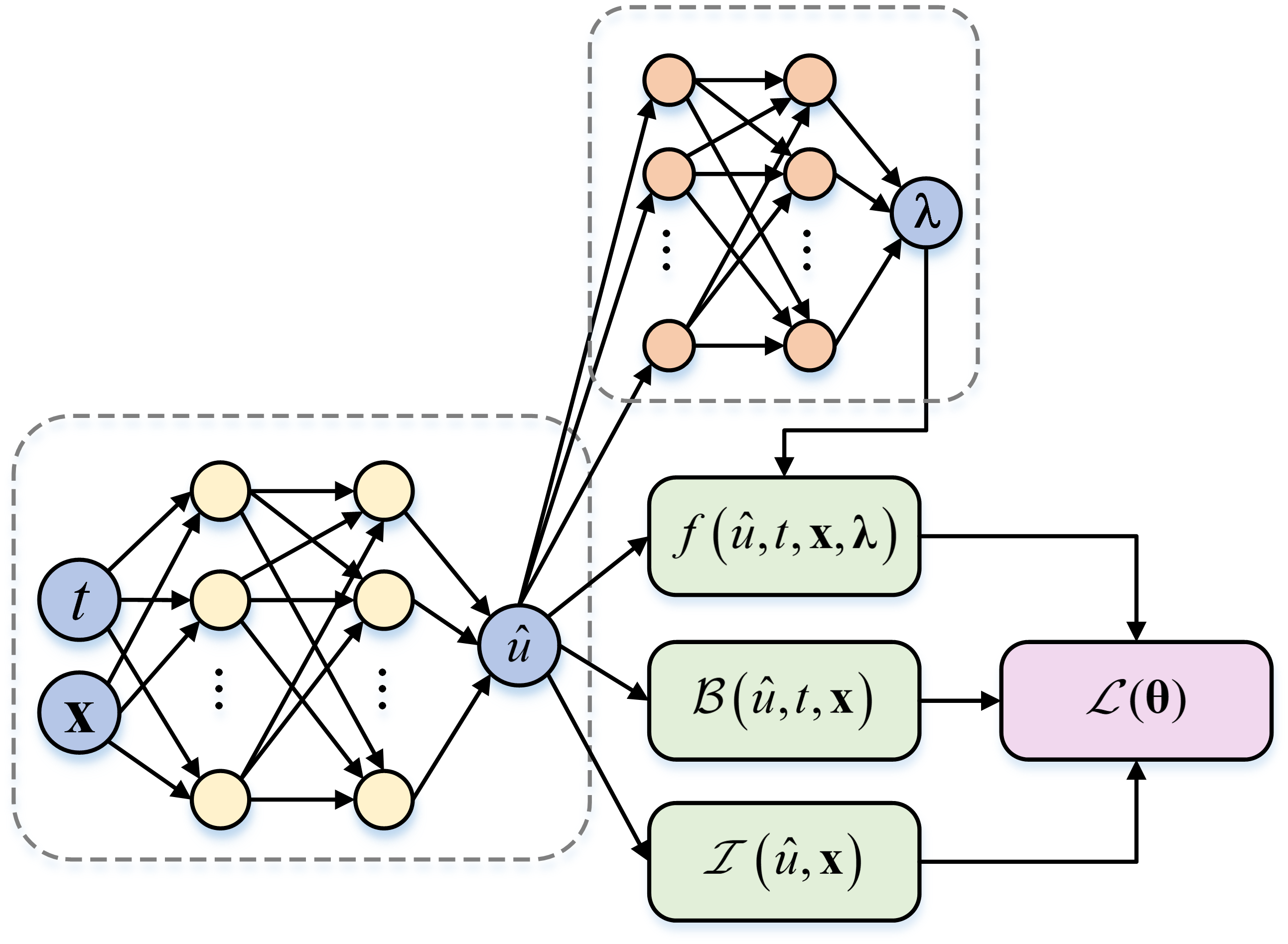Diagram of a deep neural network for thermal plasma modeling

## Case 1: stationary arc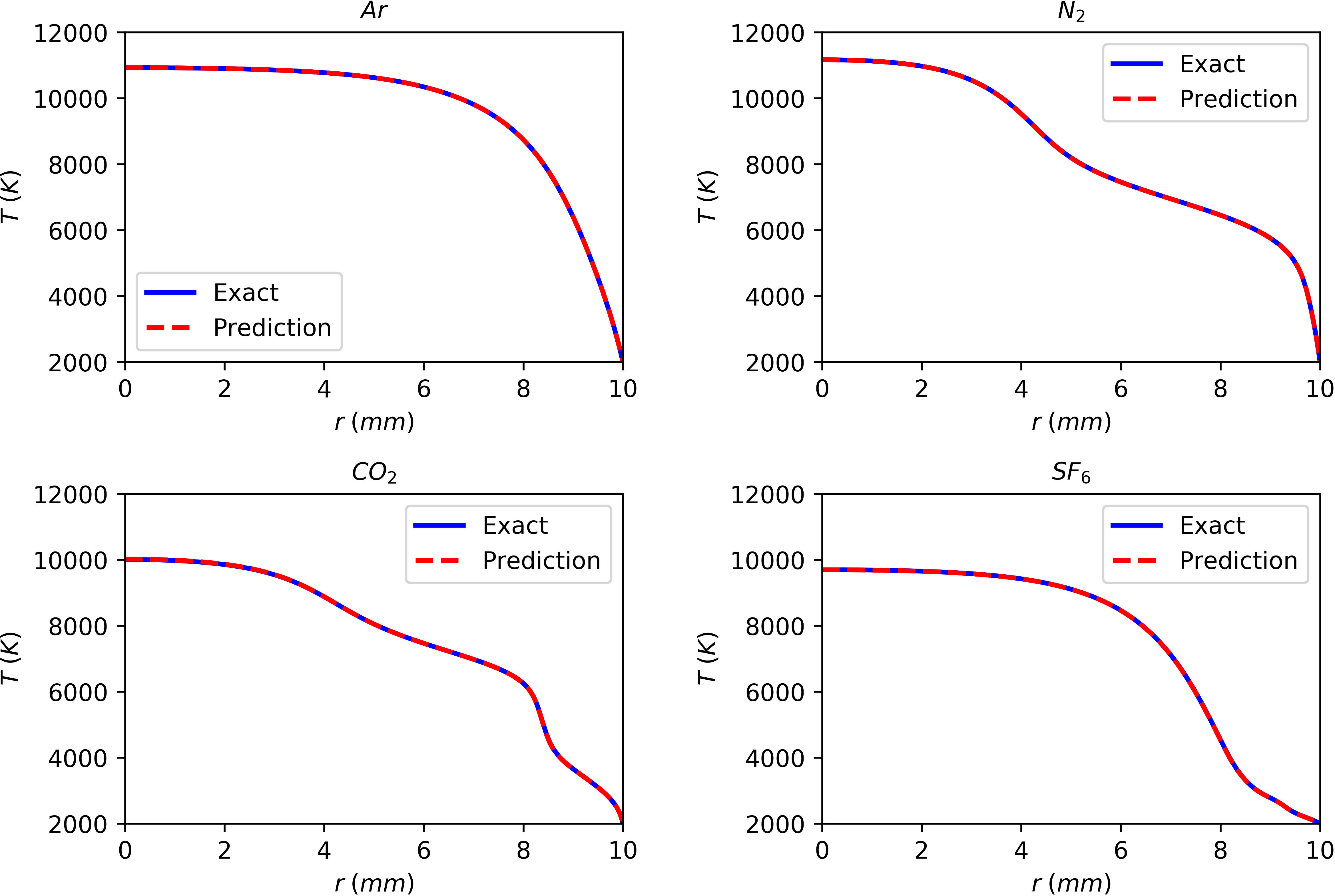Prediction of arc temperature distribution of Ar, N, CO2, and SF6 arc plasmas at ambient pressure (I = 200 A, R = 10 mm) via deep learning neural networks with comparison of the exact results by a high-order BVP solver.

## Case 2: transient arc without radial velocity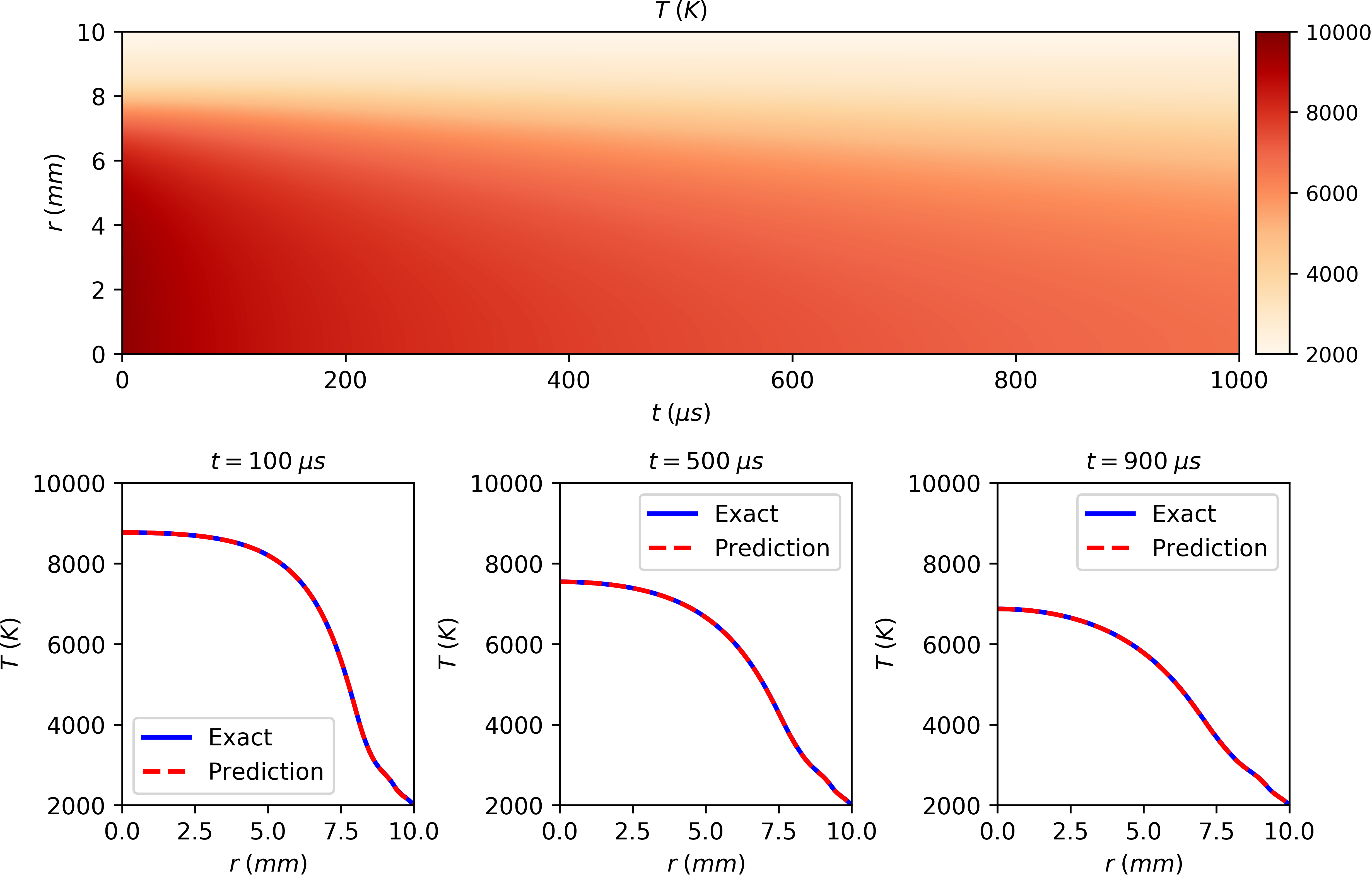Prediction of the spatial and temporal distribution of arc temperature in transient SF6 arc plasma without considering radial velocity at ambient pressure (R = 10 mm, t: 0~1000 us ) via deep learning neural networks with comparison of the exact results by an explicit method.

## Case 3: transient arc with radial velocity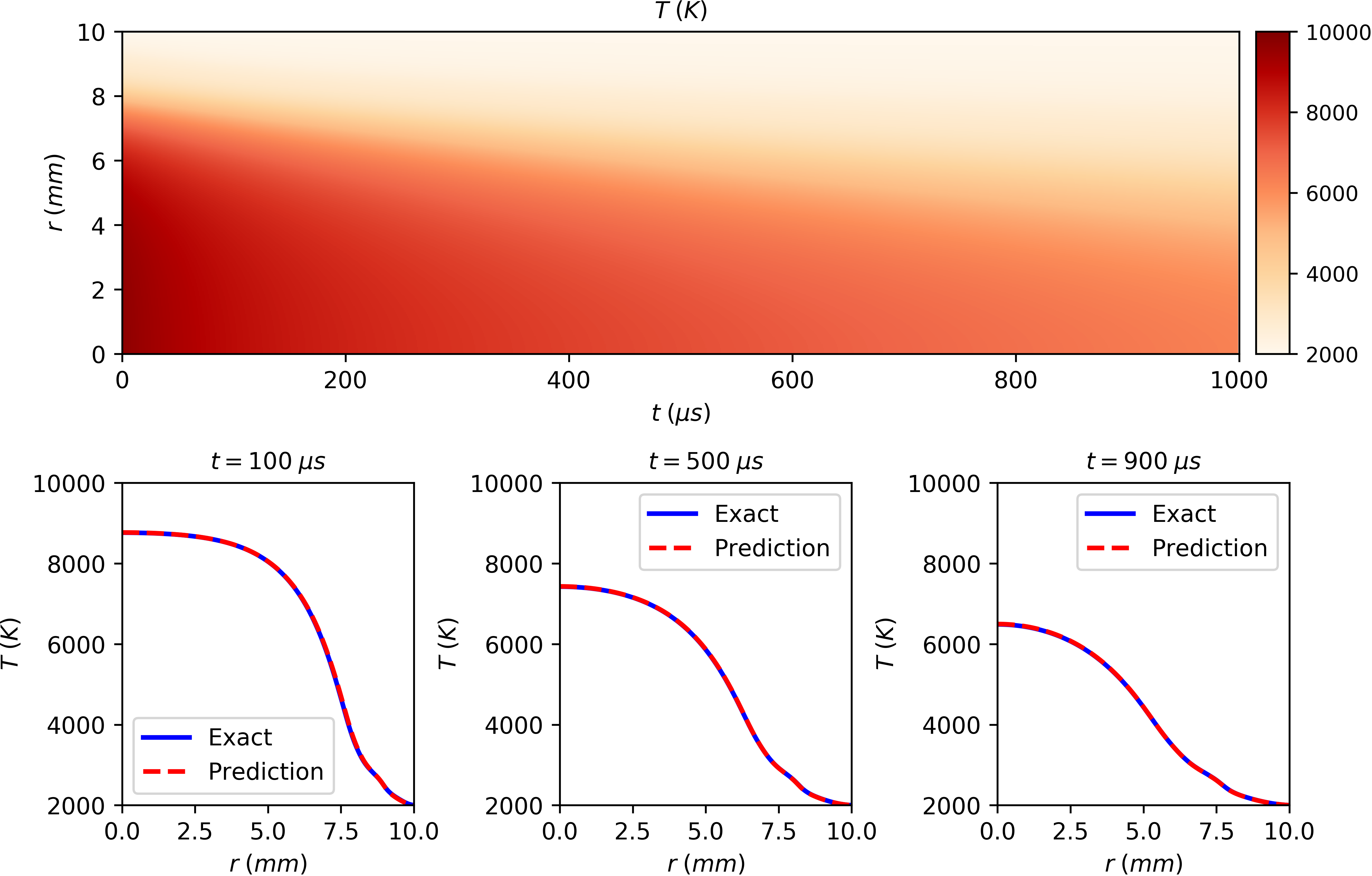Prediction of the spatial and temporal distribution of arc temperature in transient SF6 arc plasma with considering radial velocity at ambient pressure (R = 10 mm, t: 0~1000 us ) via deep learning neural networks with comparison of the exact results by an explicit method.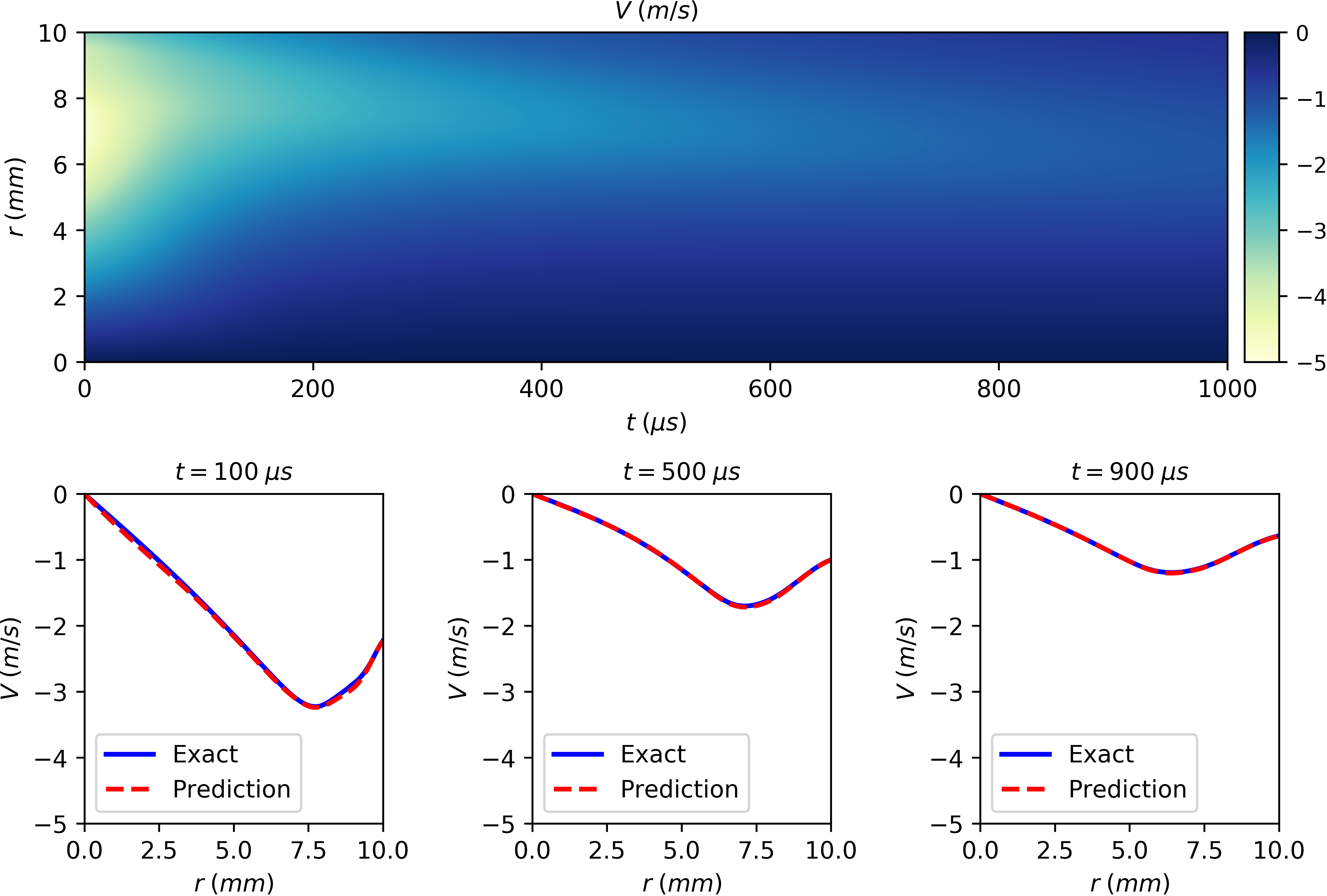Prediction of the spatial and temporal distribution of radial velocity in transient SF6 arc plasma with considering radial velocity at ambient pressure (R = 10 mm, t: 0~1000 us ) via deep learning neural networks with comparison of the exact results by an explicit method.

## Publications

. Deep learning for thermal plasma simulation: Solving 1-D arc model as an example. Computer Physics Communications, 2020.